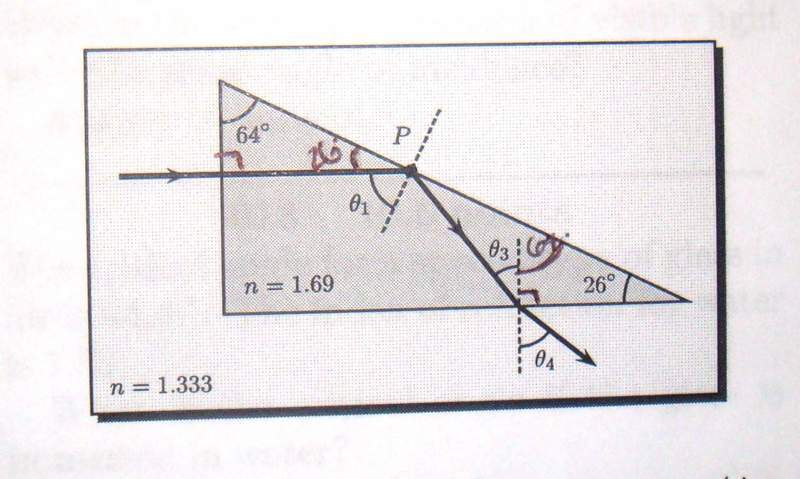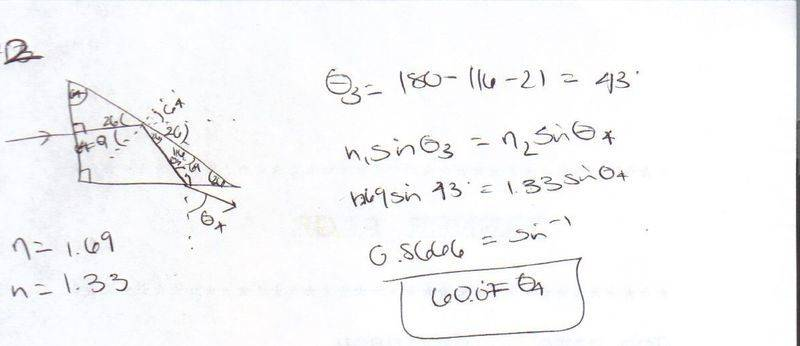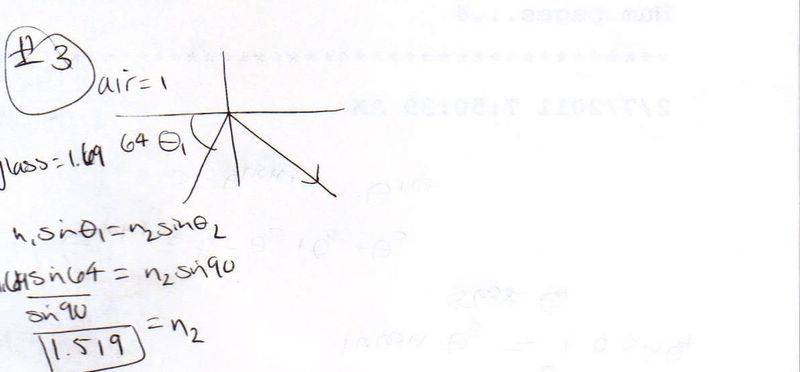# Exit angle and n2?

DrMcDreamy

## Homework Statement

This is a two part problem:

2) As shown in the ﬁgure, a light ray is incident normally on one face of a 26◦–64◦–90◦ block of dense ﬂint glass (a prism) that immersed in water. Find the exit angle θ4 of the light ray (Assume the index of grass is 1.69, and that of water is 1.333.) Answer in units of ◦.3) A substance is dissolved in the water to increase the index of refraction. At what value of n2 does total internal reﬂection cease at point P ?

## Homework Equations

n1sin$$\theta$$1=n2sin$$\theta$$2

## The Attempt at a Solution

My work:I put in the answer to number 2 and it said its wrong. What I am doing wrong? Can you guys guide me through the problem? TIA!

#### Attachments

DrMcDreamy
Anybody?!

Homework Helper
In problem 2, theta3 = 180 - (26 + 26 + 90)

DrMcDreamy
So it would be:

$$\theta$$3=180-(26+26+90)=38$$\circ$$

n3sin$$\theta$$3=n4sin$$\theta$$4
1.69sin38=1.33sin$$\theta$$4
$$\frac{1.0405}{1.33}$$=sin$$\theta$$4
sin-10.7823=$$\theta$$4
51=$$\theta$$4

And #3 is right? TIA

DrMcDreamy
*bump*

Staff Emeritus